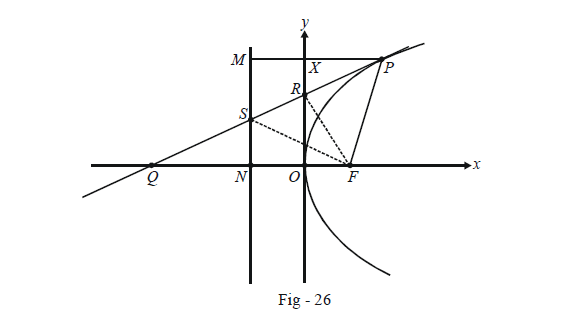# Examples on Tangents to Parabolas Set 1

Go back to  'Parabola'

Example - 17

Prove that the orthocentre of any triangle formed by three tangents to a parabola lies on its directrix.

Solution: Let the equation of the parabola be $${y^2} = 4ax$$ so that its directrix is x + a = 0. We need to show that the x-coordinate of the orthocentre is –a.

Assume any three points on the parabola as $${t_1},{\rm{ }}{t_2},{\rm{ }}{t_3}.$$ The points of intersections of the three tangents to the parabola on these three points will be

$P(a{t_1}{t_2},a({t_1} + {t_2})),\,\,Q(a{t_2}{t_3},a({t_2} + {t_3})),\,\,R(a{t_3}{t_1},a({t_3} + {t_1}))$

Let us find the equation of the altitude through P on QR.

The slope of QR is \begin{align}\frac{{a({t_2} + {t_3}) - a({t_3} + {t_1})}}{{a{t_2}{t_3} - a{t_3}{t_1}}} = \frac{1}{{{t_3}}}\end{align}

Therefore, the equation of the altitude through P is

\begin{align}&\qquad \;\;y - a({t_1} + {t_2}) = - {t_3}(x - a{t_1}{t_2})\\\\&\Rightarrow \quad y + {t_3}x = a({t_1} + {t_2} + {t_1}{t_2}{t_3}) \qquad\qquad \dots \left( 1 \right)\end{align}

By symmetry we can write the altitude from Q onto PR as

$y + {t_1}x = a({t_2} + {t_3} + {t_1}{t_2}{t_3}) \qquad\qquad\qquad \dots \left( 2 \right)$

By (1) – (2), we have

\begin{align}&\qquad \;\;({t_3} - {t_1})x = a({t_1} - {t_3})\\\\&\Rightarrow \quad x = - a\end{align}

Thus, the stated assertion is true.

Example - 18

Let P be any point on the parabola with focus F. A perpendicular PM is dropped from P onto the directrix as shown :Prove that the tangent at P to the parabola bisects the angle FPM.

Solution: Let the equation of the parabola be $${y^2} = 4ax,$$ and let P be the point t. Thus, F is the point (a, 0) while N is (–a, 0). By definition, we have

$PF = PM$

But PM is PX + XM i.e., $$a{t^2} + a.$$ Thus PF = $$a{\rm{ }} + {\rm{ }}a{t^2}$$.

Now, the equation of the tangent at P is

$ty = x + a{t^2}$

This intersects the x-axis at the point $$Q( - a{t^2},0).$$

Thus,

\begin{align}&FQ = FO + OQ\\&\quad\;\;= a + a{t^2}\end{align}

We see that in $$\Delta PFQ,$$

\begin{align}&\qquad \quad FP = FQ \\\\& \Rightarrow \quad \angle FPQ = \angle FQP \qquad \quad \dots \left( 1 \right)\end{align}

But since PM is parallel to the x -axis, we also have

$\angle FQP = \angle QPM \qquad \quad \dots \left( 2 \right)$

From (1) and (2), we obtain $$\angle FPQ = \angle QPM$$ which means that the tangent at P bisects $$\angle FPM.$$

Example – 19

Refer to Fig - 26 of the previous example. Prove that angle PFS is a right angle.

Solution: The equation of the tangent at $$P(a{t^2},2at)$$ is

$ty = x + a{t^2}$

This intersects the directrix at a point given by \left( { - a,\,\,\begin{align}\frac{{a({t^2} - 1)}}{t}\end{align}} \right)

The slope of PF is

${m_{PF}} = \frac{{2at}}{{a{t^2} - a}} = \frac{{2t}}{{{t^2} - 1}}$

The slope of SF is

{m_{SF}} = \frac{\begin{align}{\frac{{a({t^2} - 1)}}{t}}\end{align}}{{ - 2a}} = \frac{{a({t^2} - 1)}}{{ - 2t}}

Since $${m_{PF}} \times {m_{SF}} = - 1,$$ angle PFS is a right angle.